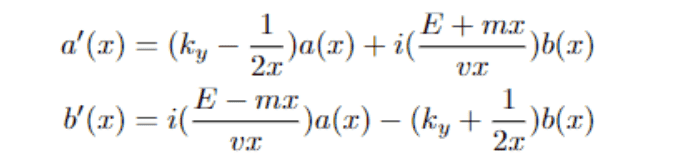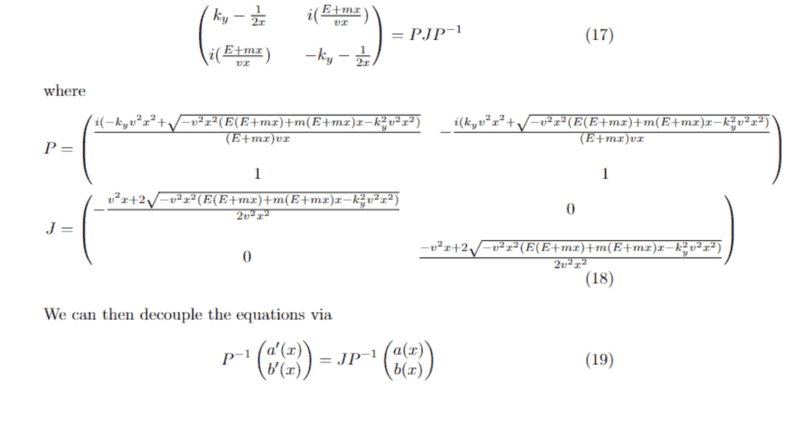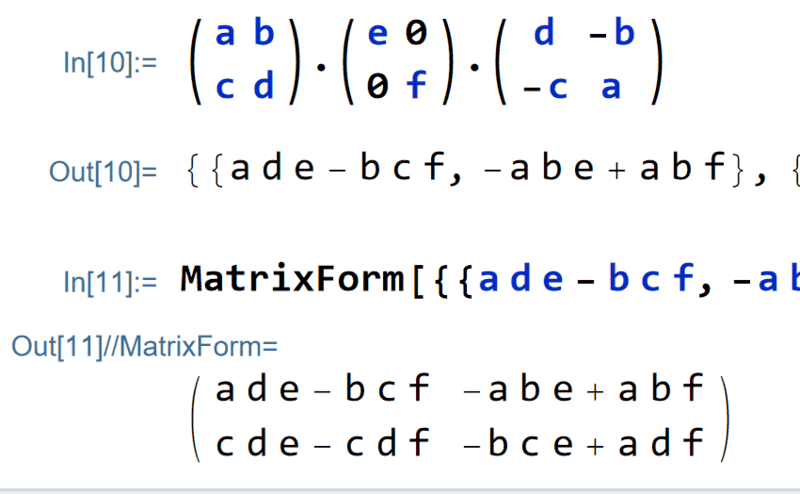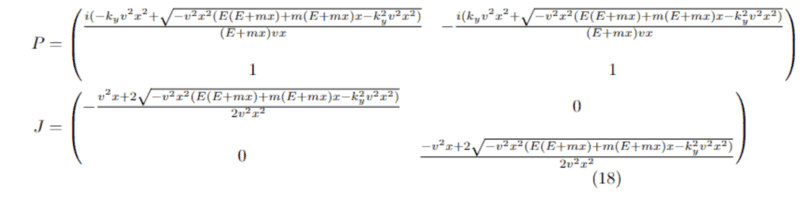# Coupled First Order ODE

• thatboi

#### thatboi

Hey all,
I am currently struggling decoupling (or just solving) a system of coupled ODEs.
The general form I wish to solve is:
a'(x)=f(x)a(x)+i*g(x)b(x)
b'(x)=i*h(x)a(x)+j(x)a(x)
where the ' indicates a derivative with respect to x, i is just the imaginary i, and f(x), g(x), h(x), and j(x) are arbitrary functions of x.
Is there a closed form solution for this kind of problem?
Any help would be appreciated.
Thanks.

•Delta2

Hey all,
I am currently struggling decoupling (or just solving) a system of coupled ODEs.
The general form I wish to solve is:
a'(x)=f(x)a(x)+i*g(x)b(x)
b'(x)=i*h(x)a(x)+j(x)a(x)
where the ' indicates a derivative with respect to x, i is just the imaginary i, and f(x), g(x), h(x), and j(x) are arbitrary functions of x.
Is there a closed form solution for this kind of problem?
Any help would be appreciated.
Thanks.
Do you have information on whether ##\begin{bmatrix}
f(x)& i g(x) \\ j(x)& ih(x)
\end{bmatrix}## is diagonalizable?

If you google "coupled differential equation system" you will probably find a lot of lecture notes. It worked at least in my language.

Do you have information on whether ##\begin{bmatrix}
f(x)& i g(x) \\ j(x)& ih(x)
\end{bmatrix}## is diagonalizable?

If you google "coupled differential equation system" you will probably find a lot of lecture notes. It worked at least in my language.
Hi,
Yes we can assume they are diagonalizable. I already tried doing a Jordan Decomposition but I would be unable to bring the derivatives outside like is usually done because my coefficient matrix is all functions of x.

It is, of course, easier if the coefficients are e.g. integers. However, the theory should be the same. We can pretend that the coefficient functions are elements of a function field, or if nothing else works, elements of the ring of formal Laurent series.

It is, of course, easier if the coefficients are e.g. integers. However, the theory should be the same. We can pretend that the coefficient functions are elements of a function field, or if nothing else works, elements of the ring of formal Laurent series.
Sorry, I do not quite follow. Could you elaborate on that or point me to examples that use these methods?

Pretend you have the solution(s) for a system with constant terms: ##\begin{bmatrix}
f & i g \\ j & ih
\end{bmatrix}##
Then look at the way it is obtained. What could have happened? Addition and multiplication aren't a problem when we substitute all constant coefficients ##c## with functions ##c(x)##. So only divisions can be problematic. But wherever we divide, we could as well use the undivided version: ##ax=b## instead of ##x=a^{-1}b##. If you need to divide the coefficient functions at all. Maybe you don't get ones on the diagonal of your normal form, so what? Or you analyze whether divisions are allowed at the points you want to use them.

And if nothing else works ##c^{-1}(x)## can probably approximated as ##c^{-1}(x)=\sum_{k=-p}^\infty c_k(x)x^k##.

Pretend you have the solution(s) for a system with constant terms: ##\begin{bmatrix}
f & i g \\ j & ih
\end{bmatrix}##
Then look at the way it is obtained. What could have happened? Addition and multiplication aren't a problem when we substitute all constant coefficients ##c## with functions ##c(x)##. So only divisions can be problematic. But wherever we divide, we could as well use the undivided version: ##ax=b## instead of ##x=a^{-1}b##. If you need to divide the coefficient functions at all. Maybe you don't get ones on the diagonal of your normal form, so what? Or you analyze whether divisions are allowed at the points you want to use them.

And if nothing else works ##c^{-1}(x)## can probably approximated as ##c^{-1}(x)=\sum_{k=-p}^\infty c_k(x)x^k##.
I am not sure if this is the same or not, but the full equations look like:where k_{y}, E, m, and v are all constants. Nevertheless, I got as far as (19) after which I am stuck because I was unable to bring the derivatives for a'(x) and b'(x) outside. Is this method still valid ultimately then?If you have ##(19)## then you are done. Multiply ##(19)## by ##\det P## and write ##\det P \cdot P^{-1}=\begin{bmatrix}
p_{22}(x)&-p_{12}(x)\\-p_{21}(x)&p_{11}(x)
\end{bmatrix}##

If you have ##(19)## then you are done. Multiply ##(19)## by ##\det P## and write ##\det P \cdot P^{-1}=\begin{bmatrix}
p_{22}(x)&-p_{12}(x)\\-p_{21}(x)&p_{11}(x)
\end{bmatrix}##
Sorry, I don't see how that solves the problem because I need to pull the derivative on the left hand side outside the product of both P^{-1} and the vector right?

\begin{align*}
P^{-1}\begin{pmatrix}
a'(x) \\ b'(x)
\end{pmatrix}&\stackrel{(19)}{=}JP^{-1}\begin{pmatrix}
a(x)\\b(x)
\end{pmatrix}\\
&\Longleftrightarrow \\
\begin{pmatrix}
a'(x) \\ b'(x)
\end{pmatrix}&=PJP^{-1}\begin{pmatrix}
a(x)\\b(x)
\end{pmatrix}\\
&\Longleftrightarrow \\
\det P\begin{pmatrix}
a'(x) \\ b'(x)
\end{pmatrix}&=
\det P \cdot
PJP^{-1}\begin{pmatrix}
a(x)\\b(x)
\end{pmatrix}\\
&\Longleftrightarrow \\
(p_{11}(x)p_{22}(x)-p_{12}(x)p_{21}(x)) \cdot
\begin{pmatrix}
a'(x) \\ b'(x)
\end{pmatrix}&=\\
\begin{pmatrix}
p_{11}(x)&p_{12}(x)\\p_{21}(x)&p_{22}(x)
\end{pmatrix}\cdot
\begin{pmatrix}
j_{11}(x)&j_{12}(x)\\j_{21}(x)&j_{22}(x)
\end{pmatrix} &\cdot
\begin{pmatrix}
p_{22}(x)&-p_{12}(x)\\-p_{21}(x)&p_{11}(x)
\end{pmatrix} \cdot
\begin{pmatrix}
a(x)\\b(x)
\end{pmatrix}
\end{align*}
This is the next step. I do not know how clumsy the factor determinant of ##P## actually is. If it does not cancel, and please beware of potential zeros(!), then you will have to consider cases. You normally do not need a global solution, so the determinant might cancel for the values of ##x## you are interested in.

Last edited:
\begin{align*}
P^{-1}\begin{pmatrix}
a'(x) \\ b'(x)
\end{pmatrix}&\stackrel{(19)}{=}JP^{-1}\begin{pmatrix}
a(x)\\b(x)
\end{pmatrix}\\
&\Longleftrightarrow \\
\begin{pmatrix}
a'(x) \\ b'(x)
\end{pmatrix}&=PJP^{-1}\begin{pmatrix}
a(x)\\b(x)
\end{pmatrix}\\
&\Longleftrightarrow \\
\det P\begin{pmatrix}
a'(x) \\ b'(x)
\end{pmatrix}&=
\det P \cdot
PJP^{-1}\begin{pmatrix}
a(x)\\b(x)
\end{pmatrix}\\
&\Longleftrightarrow \\
(p_{11}(x)p_{22}(x)-p_{12}(x)p_{21}(x)) \cdot
\begin{pmatrix}
a'(x) \\ b'(x)
\end{pmatrix}&=\\
\begin{pmatrix}
p_{11}(x)&p_{12}(x)\\p_{21}(x)&p_{22}(x)
\end{pmatrix}\cdot
\begin{pmatrix}
j_{11}(x)&j_{12}(x)\\j_{21}(x)&j_{22}(x)
\end{pmatrix} &\cdot
\begin{pmatrix}
p_{22}(x)&-p_{12}(x)\\-p_{21}(x)&p_{11}(x)
\end{pmatrix} \cdot
\begin{pmatrix}
a(x)\\b(x)
\end{pmatrix}
\end{align*}
This is the solution. I do not know how clumsy the factor determinant of ##P## actually is. If it does not cancel, and please beware of potential zeros(!), then you will have to consider cases. You normally do not need a global solution, so the determinant might cancel for the values of ##x## you are interested in.
When you say it does not cancel, what do you mean by that? From what it seems like, the system of equations have not been decoupled.

If the matrix is diagonalizable (you said it was) then there should be a diagonal matrix on the right, i.e. a decoupled version. What disturbs is the determinant on the left. We would like to divide before integration. But this depends on whether we are allowed to.

If the matrix is diagonalizable (you said it was) then there should be a diagonal matrix on the right, i.e. a decoupled version. What disturbs is the determinant on the left. We would like to divide before integration. But this depends on whether we are allowed to.
But just referring to any (suppose diagonalizable) 2x2 matrix, I get the following:Are you claiming that this matrix is diagonal?

A matrix ##A## is diagonalizable if there is a regular matrix ##P## such that ##P^{-1}AP=D## is a diagonal matrix ##D##. This is more or less the same as saying the equations can be decoupled. The art is to find ##P##, and since we have functions as coefficients, we must do so without divisions. But this can be done if we carry the determinant all the way because this is the only term that needs to be divided.

Have you checked the characteristic polynomial of your coefficient matrix? At a first glimpse, it looks as if that matrix is indeed diagonalizable with all those symmetries and conjugates. You should get two one-dimensional Jordan blocks, i.e. ##J## should be a diagonal matrix.

A matrix ##A## is diagonalizable if there is a regular matrix ##P## such that ##P^{-1}AP=D## is a diagonal matrix ##D##. This is more or less the same as saying the equations can be decoupled. The art is to find ##P##, and since we have functions as coefficients, we must do so without divisions. But this can be done if we carry the determinant all the way because this is the only term that needs to be divided.

Have you checked the characteristic polynomial of your coefficient matrix? At a first glimpse, it looks as if that matrix is indeed diagonalizable with all those symmetries and conjugates. You should get two one-dimensional Jordan blocks, i.e. ##J## should be a diagonal matrix.
Yes, indeed that is the case, so (17) is the equation that I performed the Jordan decomposition on, i.e J is diagonal and P is the matrix that satisfies (17). With that being said, doing det(P)*P*J*P^{-1} should not be diagonal in my mind because it is simply multiplying the LHS of (17) by a constant factor right?
(18) gives the explicit form of the decomposition if that helps.

The determinant is ##\det P=2is## where ##s## is the root expression (and denominator). Since ##v,x\neq 0## anyway, we will have no problems with the determinant. This means you can proceed with the literature that normally deals with numbers as coefficients.

Introduce new variables:
\begin{align*}
P^{-1}(x)&:=
\dfrac{1}{(p_{11}(x)p_{22}(x)-p_{12}(x)p_{21}(x))}\cdot \begin{pmatrix}
p_{22}(x)&-p_{12}(x)\\-p_{21}(x)&p_{11}(x)
\end{pmatrix}\cdot
\begin{pmatrix}
a(x)\\b(x)
\end{pmatrix} \\
A(x)&:= \dfrac{1}{(p_{11}(x)p_{22}(x)-p_{12}(x)p_{21}(x))}\cdot (p_{22}(x)a(x)-p_{12}b(x))\\
B(x)&:= \dfrac{1}{(p_{11}(x)p_{22}(x)-p_{12}(x)p_{21}(x))}\cdot (-p_{21}(x)a(x)+p_{11}b(x))
\end{align*}
Now we have new decoupled variables ##A(x)## and ##B(x)##.

The determinant is ##\det P=2is## where ##s## is the root expression (and denominator). Since ##v,x\neq 0## anyway, we will have no problems with the determinant. This means you can proceed with the literature that normally deals with numbers as coefficients.

Introduce new variables:
\begin{align*}
P^{-1}(x)&:=
\dfrac{1}{(p_{11}(x)p_{22}(x)-p_{12}(x)p_{21}(x))}\cdot \begin{pmatrix}
p_{22}(x)&-p_{12}(x)\\-p_{21}(x)&p_{11}(x)
\end{pmatrix}\cdot
\begin{pmatrix}
a(x)\\b(x)
\end{pmatrix} \\
A(x)&:= \dfrac{1}{(p_{11}(x)p_{22}(x)-p_{12}(x)p_{21}(x))}\cdot (p_{22}(x)a(x)-p_{12}b(x))\\
B(x)&:= \dfrac{1}{(p_{11}(x)p_{22}(x)-p_{12}(x)p_{21}(x))}\cdot (-p_{21}(x)a(x)+p_{11}b(x))
\end{align*}
Now we have new decoupled variables ##A(x)## and ##B(x)##.
Sorry, I must not be seeing something very obvious, are you saying that with the P^{-1}(x) and A(x) and B(x) as you have written them, (19) will now become decoupled? I somehow do not think the derivatives of the A(x) and B(x) will not work out as nicely.

Sorry, I must not be seeing something very obvious, are you saying that with the P^{-1}(x) and A(x) and B(x) as you have written them, (19) will now become decoupled? I somehow do not think the derivatives of the A(x) and B(x) will not work out as nicely.

You are right, one has to be a bit more careful. I have fallen into the pit of the ordinary case where we have a static linear transformation. I'll try to figure it out, but I will have to use paper. Maybe the constants in ##P(x)## are of help.

##P(x)## isn't a differential at a certain point by chance?

You are right, one has to be a bit more careful. I have fallen into the pit of the ordinary case where we have a static linear transformation. I'll try to figure it out, but I will have to use paper. Maybe the constants in ##P(x)## are of help.

##P(x)## isn't a differential at a certain point by chance?
For reference, P(x) and J(x) are here:I do not think P(x) is a differential at any point though.

For reference, P(x) and J(x) are here:
View attachment 302833
I do not think P(x) is a differential at any point though.
Don't mind, we do not need it. I'm currently typing it ...

Let's go. I make the following settings so that I will not have to type so many letters, but I will keep the dependencies on ##x## for not falling into the same trap again:
\begin{align*}
J(x)&=\begin{bmatrix}
\mu(x) & 0 \\0 & \lambda(x)
\end{bmatrix}\, , \,P:=\begin{bmatrix}
\alpha(x) &\beta(x) \\1&1
\end{bmatrix}\, , \,\det P(x)=2\cdot i \cdot s(x)=\alpha(x)-\beta (x)\\
\end{align*}
Then we multiply ##(19)## by ##\det P## on both sides. Thus we get from ##(19)##
\begin{align*}
\det P(x) P^{-1}(x)\begin{bmatrix}
a'(x) \\ b'(x)
\end{bmatrix} &= \begin{bmatrix}
1& -\beta(x) \\ -1& \alpha(x)
\end{bmatrix} \cdot \begin{bmatrix}
a'(x) \\ b'(x)
\end{bmatrix}=\begin{bmatrix}
a'(x)-\beta(x)b'(x) \\ -a'(x)+\alpha(x)b'(x)
\end{bmatrix} \\
&\stackrel{(19)}{=} \det P(x) J(x) P^{-1}(x)\begin{bmatrix}
a(x) \\b(x)
\end{bmatrix} =\ldots\\
&=\ldots = J\cdot
\begin{bmatrix}
a(x)-\beta(x)b(x) \\ -a(x)+\alpha(x)b(x)
\end{bmatrix}=
\begin{bmatrix}
\mu(x) a(x)-\mu(x)\beta(x)b(x) \\ -\lambda (x)a(x)+\lambda (x)\alpha(x)b(x)
\end{bmatrix}
\end{align*}
Now we have
\begin{align*}
\begin{bmatrix}
a'(x)-\beta(x)b'(x) \\ -a'(x)+\alpha(x)b'(x)
\end{bmatrix}=
\begin{bmatrix}
\mu(x) a(x)-\mu(x)\beta(x)b(x) \\ -\lambda (x)a(x)+\lambda (x)\alpha(x)b(x)
\end{bmatrix}
\end{align*}
Next set ##A(x):=a(x)-\beta(x)b(x)## and ##B(x):=-a(x)+\alpha(x)b(x).## This yields
\begin{align*}
\mu(x)A(x)&=A'(x) +\beta'(x)b(x)\\
\lambda(x)B(x)&=B'(x)-\alpha'(x)a(x)
\end{align*}
or
\begin{align*}
A'(x)&= \mu(x)A(x)-\beta'(x)b(x)\\
B'(x)&=\lambda(x)B(x)+\alpha'(x)a(x)
\end{align*}
an inhomgeneous linear system.
(I apologize for typos ...)

Last edited:
Let's go. I make the following settings so that I will not have to type so many letters, but I will keep the dependencies on ##x## for not falling into the same trap again:
\begin{align*}
J(x)&=\begin{bmatrix}
\mu(x) & 0 \\0 & \lambda(x)
\end{bmatrix}\, , \,P:=\begin{bmatrix}
\alpha(x) &\beta(x) \\1&1
\end{bmatrix}\, , \,\det P(x)=2\cdot i \cdot s(x)=\alpha(x)-\beta (x)\\
\end{align*}
Then we multiply ##(19)## by ##\det P## on both sides. Thus we get from ##(19)##
\begin{align*}
\det P(x) P^{-1}(x)\begin{bmatrix}
a'(x) \\ b'(x)
\end{bmatrix} &= \begin{bmatrix}
1& -\beta(x) \\ -1& \alpha(x)
\end{bmatrix} \cdot \begin{bmatrix}
a'(x) \\ b'(x)
\end{bmatrix}=\begin{bmatrix}
a'(x)-\beta(x)b'(x) \\ -a'(x)+\alpha(x)b'(x)
\end{bmatrix} \\
&\stackrel{(19)}{=} \det P(x) J(x) P^{-1}(x)\begin{bmatrix}
a(x) \\b(x)
\end{bmatrix} =\ldots\\
&=\ldots = J\cdot
\begin{bmatrix}
a(x)-\beta(x)b(x) \\ -a(x)+\alpha(x)b(x)
\end{bmatrix}=
\begin{bmatrix}
\mu(x) a(x)-\mu(x)\beta(x)b(x) \\ -\lambda (x)a(x)+\lambda (x)\alpha(x)b(x)
\end{bmatrix}
\end{align*}
Now we have
\begin{align*}
\begin{bmatrix}
a'(x)-\beta(x)b'(x) \\ -a'(x)+\alpha(x)b'(x)
\end{bmatrix}=
\begin{bmatrix}
\mu(x) a(x)-\mu(x)\beta(x)b(x) \\ -\lambda (x)a(x)+\lambda (x)\alpha(x)b(x)
\end{bmatrix}
\end{align*}
Next set ##A(x):=a(x)-\beta(x)b(x)## and ##B(x):=-a(x)+\alpha(x)b(x).## This yields
\begin{align*}
\mu(x)A(x)&=A'(x) +\beta'(x)b(x)\\
\lambda(x)B(x)&=B'(x)-\alpha'(x)a(x)
\end{align*}
or
\begin{align*}
A'(x)&= \mu(x)A(x)-\beta'(x)b(x)\\
B'(x)&=\lambda(x)B(x)+\alpha'(x)a(x)
\end{align*}
an inhomgeneous linear system.
(I apologize for typos ...)
Hmm, so what it seems is that there is no way to completely decouple the system?

Hmm, so what it seems is that there is no way to completely decouple the system?
We are done. At least in principle. This method
https://en.wikipedia.org/wiki/Variation_of_parameters#First-order_equation
solves for ##A(x)## and ##B(x)## and with them we have ##a(x)## and ##b(x)## by solving another linear equation system.

Decoupling is possible if diagonalizable. E.g. hermitian matrices are always diagonalizable with unitarian matrices.

Last edited:
•thatboi
We are done. At least in principle. This method
https://en.wikipedia.org/wiki/Variation_of_parameters#First-order_equation
solves for ##A(x)## and ##B(x)## and with them we have ##a(x)## and ##b(x)## by solving another linear equation system.

Decoupling is possible if diagonalizable. E.g. hermitian matrices are always diagonalizable with unitarian matrices.
Alright this all makes sense and I was mostly able to work out a solution of sorts, thank you so much! I just need to chew on the actual expression itself and hopefully make some sense out of it. Thank you again for such prompt responses.

•fresh_42 and jim mcnamara
Next set ##A(x):=a(x)-\beta(x)b(x)## and ##B(x):=-a(x)+\alpha(x)b(x).##
This yields

\begin{align*}
A'(x)&= \mu(x)A(x)-\beta'(x)b(x)\\
B'(x)&=\lambda(x)B(x)+\alpha'(x)a(x)
\end{align*}
an inhomgeneous linear system.
(I apologize for typos ...)
Sorry, I don’t see how this solves anything. You introduced ##A(x)## and ##B(x)## as linear combinations of ##a(x)## and ##b(x)##, which is what you are attempting to solve for. You therefore cannot leave ##a(x)## and ##b(x)## as known inhomogeneties in the DEs. The inverse relation is (suppressing the x-depenence to write less)
$$\alpha A + \beta B = (\alpha -\beta) a.$$
Inserting this into the differential equation for ##B## yields
$$B’(x) = \lambda(x) B(x) +\alpha’(x) \frac{\alpha(x)A(x) + \beta(x) B(x)}{\alpha(x) -\beta(x)}.$$
Therefore, the differential equation for ##B(x)## does not decouple and a similar argument holds for ##A(x)##.

Edit: The reason I am familiar with this type of problem is that it appears in the mathematics of quantum two-level systems with time-varying Hamiltonian. This is particularly applicable to two-flavour neutrino oscillations in an arbitrary matter density profile. In some cases ##\alpha’## may be considered small, thus essentially decoupling the eigenstates. This is known as the adiabatic approximation and is useful to describe solar neutrinos. However, no closed form exact solution for the general problem is known.

Last edited:
But then the solution ##A(x)## depends on ##b(x)## (and ##B(x)## on ##a(x)##) in a non-trivial way and you cannot use the linear equation to find ##a(x)## and ##b(x)##.
Yes, you are right. I thought it was easier. Looks as if ##\alpha'=\beta'=0## as in the usual situation cannot be omitted easily.

Is there a decoupling from a physical point of view?

Yes, you are right. I thought it was easier. Looks as if ##\alpha'=\beta'=0## as in the usual situation cannot be omitted easily.

Is there a decoupling from a physical point of view?
I made an edit to my first post regarding why it is important in my field, I don’t know if you saw it. Approximating the non-diagonal term to zero is a good approximation in some cases (such as solar neutrinos - I think the probability of switching eigenstate is approximately 1e-500 iirc) but will fail miserably in others.

•fresh_42